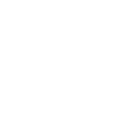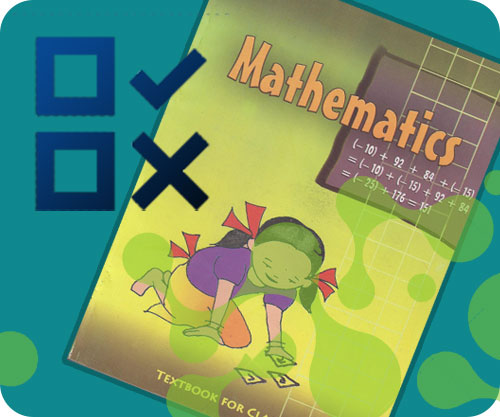×### 6th class Math Chapter Wise Online Test## 6th Class Mathematics MCQ Test With Answer for Mathematics Full Book

#### 6th Class Math Full Book Test

Start Full Book Test

### 6th Class Mathematics MCQ Test With Answer for Mathematics Full Book

1 A number line is a straight line on which each point represent a.
• A. Number
• B. Square
• C. Square root
• D. Absolute value
2 A variable whose value is obtained by measurement is called.
• A. Discrete variable
• B. Continous variable
• C. Both a and b
• D. None
3 Any given number is even if the digit on its ones place is multiple of.
• A. 1
• B. 2
• C. 3
• D. 4
4 Types of bar graph are.
• A. One
• B. Two
• C. Three
• D. Four
5 A sphere is a 3- dimensional solid object, it has.
• A. 6 surfaces , 12 edges , 8 vertices.
• B. 0 surfaces, 0 edges , 1 verties
• C. 0 surfaces, 0 edges, 0 vertices
• D. 6 surfaces, 2 edges, 0 vertices.
6 The mess is also known as.
• A. Speed
• B. Distance
• D. Average
7 There are ...... vertices of cylinder.
• A. Four
• B. Three
• C. Two
• D. No
8 Area of parallelogram is same as area.
• A. Rectangle
• B. Square
• C. Triangle
• D. Circle
9 A polygon is a closed shpe which has......... straight edges.
• A. One
• B. Two
• C. Three
• D. Three or more
10 A point where two lines intreesect each other is called.
• A. Corner point
• B. Centre point
• C. Point of intersect
• D. None of these

Sort By:
• S

15 May 2019

good

Like
• A

#### Ali

03 Oct 2018

good

Like (3)
X

to continue to ilmkidunya.comFill the form. Our admission consultants will call you with admission options.

X

to continue to ilmkidunya.com

X

to continue to ilmkidunya.com

X